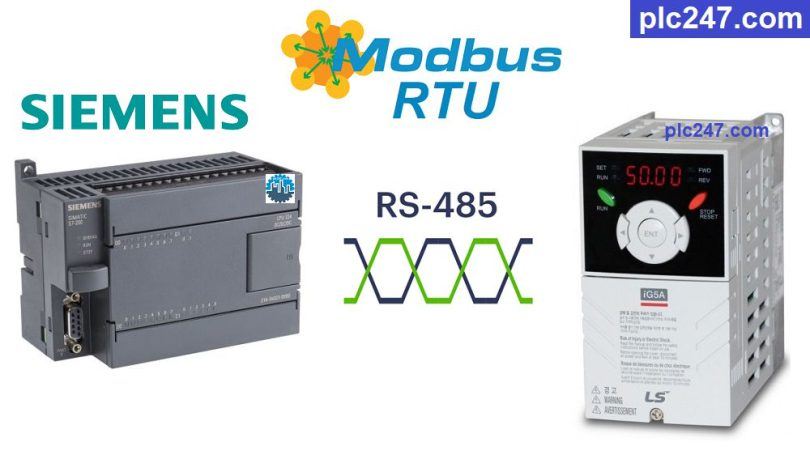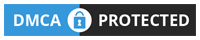# Siemens S7-200 “Modbus RTU” LS iG5A InverterWritten by

Hello friends!
Continuing the series of articles on how to use Siemens PLC to control different devices through Modbus RTU, today plc247.com will guide you to control the LS iG5A Inverter.

Both of these devices have built-in RS485 communication, plc247.com will guide you to connect and program so they can connect to each other in the simplest and easiest way.

#### Siemens S7-200 & LS iG5A “Connection Diagram”

Below is the RS485 communication connection diagram between Siemens S7-200 and LS iG5A Inverter.

#### LS iG5A “Modbus RTU” Parameters Setting

To be able to control the LS iG5A inverter with Modbus-RTU, in addition to the basic parameters of the inverter (frequency, voltage, current …) we need to install the following communication parameters:

plc247.com recommends that you install the following for stable communication:

• Drv = 3
• Frq = 7
• I59 = 0 (Choose Modbus-RTU)
• I60 = 1 (Slave No.1)
• I61 = 3 (baudrate 9600bps)
• I62 = 0
• I65 = 2 (Even/1bit)
##### Note: Siemens S7-200 & LS iG5A Holding Registers Address = “VFD Address (Dec) + 40000“

+ Frequency Setting Address = 5 + 40000 = 40005 (dec)

+ VFD Control Address = 6 + 40000 = 40006 (dec)

• Set Word “40006” = 2 >>> Run Forward
• Set Word “40006” = 4 >>> Run Reverse
• Set Word “40006” = 0 >>> Stop

+ Current Output Address = 9 + 40000 = 40009 (dec)
+ Frequency Output Address = A (hex) + 40000 = 10 (dec) + 40000 = 40010 (dec)
+ Voltage Output Address = B (hex) + 40000 = 11 (dec) + 40000 = 40011 (dec)

### PLC Programming Example

+ Modbus Parameters Configuration

+ Frequency Setting Write

+ VFD Control Run

### Project Video Tutorial

======

###### Related Software and Documents